CBSE Board NCERT Solutions for Class 10th Mathematics Chapter 12 Areas related to Circles
CBSE NCERT Solutions for Class Ten Mathematics Chapter 12 Areas related to Circles
NCERT Solutins For Class 10 Mathematics. Exercise 12.1, Exercise 12.2, Exercise 12.3.

NCERT Solutions for Class X Maths Chapter 12 Areas Related To Circles – Mathematics CBSE
Page No: 230
Exercise: 12.1

Unless stated otherwise, use π =22/7.
1. The radii of two circles are 19 cm and 9 cm respectively. Find the radius of the circle which has circumference equal to the sum of the circumferences of the two circles.
Let the radius of the third circle be R.
Circumference of the circle with radius R = 2πR
Circumference of the circle with radius 19 cm = 2π × 19 = 38π cm
Circumference of the circle with radius 9 cm = 2π × 9 = 18π cm
Sum of the circumference of two circles = 38π + 18π = 56π cm
Circumference of the third circle = 2πR = 56π
⇒ 2πR = 56π cm
⇒ R = 28 cm
The radius of the circle which has circumference equal to the sum of the circumferences of the two circles is 28 cm.
2.  The radii of two circles are 8 cm and 6 cm respectively. Find the radius of the circle having area equal to the sum of the areas of the two circles.
Area of the circle with radius R = πR2
Area of the circle with radius 8 cm = π × 82 = 64π cm2
Area of the circle with radius 6 cm = π × 62 = 36π cm2
Sum of the area of two circles = 64π cm2 + 36π cm2 = 100π cm2
Area of the third circle = πR2 = 100π cm2
⇒ πR2 = 100π cm2
⇒ R2 = 100 cm2
⇒ R = 10 cm
Thus, the radius of the new circle is 10 cm.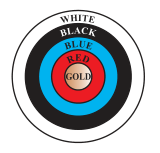3. Fig. 12.3 depicts an archery target marked with its five scoring regions from the centre outwards as Gold, Red, Blue, Black and White. The diameter of the region representing Gold score is 21 cm and each of the other bands is 10.5 cm wide. Find the area of each of the five scoring regions.
Diameter of Gold circle (first circle) = 21 cm
Radius of first circle, r1 = 21/2 cm = 10.5 cm
Each of the other bands is 10.5 cm wide,
∴ Radius of second circle, r2 = 10.5 cm + 10.5 cm = 21 cm
∴ Radius of third circle, r3 = 21 cm + 10.5 cm = 31.5 cm
∴ Radius of fourth circle, r4 = 31.5 cm + 10.5 cm = 42 cm
∴ Radius of fifth circle, r5 = 42 cm + 10.5 cm = 52.5 cm
Area of gold region = π r12 = π (10.5)2 = 346.5 cm2
Area of red region = Area of second circle – Area of first circle = π r22 – 346.5 cm2

= π(21)2 – 346.5 cm2 = 1386 – 346.5 cm2 = 1039.5 cm2
Area of blue region = Area of third circle – Area of second circle = π r32 – 1386 cm2

=  π(31.5)2 – 1386 cm2 = 3118.5 – 1386 cm2 = 1732.5 cm2
Area of black region = Area of fourth circle – Area of third circle = π r32 – 3118.5 cm2

= π(42)2 – 1386 cm2 = 5544 – 3118.5 cm2 = 2425.5 cm2
Area of white region = Area of fifth circle – Area of fourth circle = π r42 – 5544 cm2

= π(52.5)2 – 5544 cm2 = 8662.5 – 5544 cm2 = 3118.5 cm2

4. The wheels of a car are of diameter 80 cm each. How many complete revolutions does each wheel make in 10 minutes when the car is travelling at a speed of 66 km per hour?
Answer : Diameter of the wheels of a car = 80 cm
Circumference of wheels = 2πr = 2r × π = 80 π cm
Distance travelled by car in 10 minutes = (66 × 1000 × 100 × 10)/60 = 1100000 cm/s
No. of revolutions = Distance travelled by car/Circumference of wheels
= 1100000/80 π = (1100000 × 7)/(80×22) = 4375
4375 complete revolutions does each wheel make in 10 minutes.
5. Tick the correct answer in the following and justify your choice : If the perimeter and the area of a circle are numerically equal, then the radius of the circle is
(A) 2 units                     (B) π units                  (C) 4 units              (D) 7 units
∴ Perimeter of the circle = Circumference of the circle = 2πr
∴ Area of the circle = π r2
A/q,

2πr = π r2
⇒ 2 = r
Thus, the radius of the circle is 2 units. (A) is correct.

Page No: 230
Exercise: 12.2

Unless stated otherwise, use π =22/7.
1. Find the area of a sector of a circle with radius 6 cm if angle of the sector is 60°.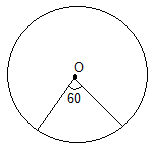Answer : Area of the sector making angle θ = (θ/360°)×π r2
Area of the sector making angle 60° = (60°/360°)×π r2 cm2
= (1/6)×62π  = 36/6 π cm2 = 6 × 22/7 cm2 = 132/7 cm2

2. Find the area of a quadrant of a circle whose circumference is 22 cm.

Answer : Quadrant of a circle means sector is making angle 90°.
Circumference of the circle = 2πr = 22 cm
Radius of the circle = r = 22/2π cm = 7/2 cm
Area of the sector making angle 90° = (90°/360°)×π r2 cm2
= (1/4)×(7/2)2π  = (49/16) π cm2 = (49/16) × (22/7) cm2 = 77/8 cm2

3. The length of the minute hand of a clock is 14 cm. Find the area swept by the minute hand in 5 minutes.
Answer : Here, Minute hand of clock acts as radius of the circle.
∴ Radius of the circle (r) = 14 cm
Angle rotated by minute hand in 1 hour = 360°
∴ Angle rotated by minute hand in 5 minutes = 360° × 5/60 = 30°
Area of the sector making angle 30° = (30°/360°)×π r2 cm2
= (1/12) × 142π  = 196/12 π cm2 = (49/3) × (22/7) cm2 = 154/3 cm2
Area swept by the minute hand in 5 minutes = 154/3 cm2

4. A chord of a circle of radius 10 cm subtends a right angle at the centre. Find the area of the corresponding : (i) minor segment (ii) major sector. (Use π = 3.14)

Radius of the circle = 10 cm
Major segment is making 360° – 90° = 270°
Area of the sector making angle 270°
= (270°/360°) × π r2 cm2
= (3/4) × 102π  = 75 π cm2
= 75 × 3.14 cm2 = 235.5 cm2
∴ Area of the major segment = 235.5 cm2
Height of ΔAOB = OA = 10 cm
Base of ΔAOB = OB = 10 cm
Area of ΔAOB = 1/2 × OA × OB
= 1/2 ×10 × 10 = 50 cm2
Major segment is making  90°
Area of the sector making angle 90°                                                                = (90°/360°) × π r2 cm2
= (1/4) × 102π  = 25 π cm2
= 25 × 3.14 cm2 = 78.5 cm2
Area of the minor segment = Area of the sector making angle 90° – Area of ΔAOB
= 78.5 cm2 –  50 cm2 = 28.5 cm2

5. In a circle of radius 21 cm, an arc subtends an angle of 60° at the centre. Find:
(i) the length of the arc
(ii) area of the sector formed by the arc
(iii) area of the segment formed by the corresponding chord

Radius of the circle = 21 cm
(i) Length of the arc AB = θ/360° × 2πr
= 60°/360° × 2 × 22/7 × 21
= 1/6 × 2 × 22/7 × 21 = 22
The length of the arc is  22 cm.
(ii) Angle subtend by the arc = 60°
Area of the sector making angle 60° = (60°/360°) × π r2 cm2
= (1/6) × 212π  = 441/6 π cm2
= 441/6 × 22/7 cm2 = 231 cm2
∴ Area of the sector formed by the arc is 231 cm2

(iii) Area of equilateral ΔAOB = √3/4 × (OA)2 = √3/4 × 212 = (441√3)/4 cm2
Area of the segment formed by the corresponding chord
= Area of the sector formed by the arc – Area of equilateral ΔAOB
= 231 cm2 – (441√3)/4 cm2

6. A chord of a circle of radius 15 cm subtends an angle of 60° at the centre. Find the areas of the corresponding minor and major segments of the circle. (Use π = 3.14 and √3 = 1.73)

Radius of the circle = 15 cm
ΔAOB is isosceles as two sides are equal.
∴ ∠A = ∠B
Sum of all angles of triangle = 180°
∠A + ∠B + ∠C = 180°
⇒ 2 ∠A = 180° – 60°
⇒ ∠A = 120°/2
⇒ ∠A = 60°
Triangle is equilateral as ∠A = ∠B = ∠C = 60°
∴ OA = OB = AB = 15 cm
Area of equilateral ΔAOB = √3/4 × (OA)2 = √3/4 × 152
= (225√3)/4 cm2 = 97.3 cm2
Angle subtend at the centre by minor segment = 60°
Area of Minor sector making angle 60° = (60°/360°) × π r2 cm2
= (1/6) × 152 π  cm2 =  225/6 π  cm2
=  (225/6) × 3.14 cm2 = 117.75  cm2

Area of the minor segment = Area of Minor sector – Area of equilateral ΔAOB

= 117.75  cm2 – 97.3 cm2 = 20.4 cm2
Angle made by Major sector = 360° – 60° = 300°
Area of the sector making angle 300° = (300°/360°) × π r2 cm2
= (5/6) × 152 π  cm2 =  1125/6 π  cm2
=  (1125/6) × 3.14 cm2 = 588.75  cm2

Area of major segment = Area of Minor sector + Area of equilateral ΔAOB

= 588.75  cm2 + 97.3 cm2 = 686.05 cm2

7. A chord of a circle of radius 12 cm subtends an angle of 120° at the centre. Find the area of the corresponding segment of the circle. (Use π = 3.14 and √3 = 1.73)

Radius of the circle, r = 12 cm
Draw a perpendicular OD to chord AB. It will bisect AB.
∠A = 180° – (90° + 60°) = 30°
⇒ AB = 2 × AD = 12√3 cm
sin 30° = OD/OA
⇒ 1/2 = OD/12
⇒ OD = 6 cm
Area of ΔAOB = 1/2 × base × height
= 1/2 × 12√3 × 6 = 36√3 cm
= 36 × 1.73 = 62.28 cm2

Angle made by Minor sector = 120°

Area of the sector making angle 120° = (120°/360°) × π r2 cm2
= (1/3) × 122 π  cm2 =  144/3 π  cm2
=  48 × 3.14 cm2 = 150.72  cm2
∴ Area of the corresponding Minor segment = Area of the Minor sector – Area of ΔAOB
= 150.72  cm2 – 62.28 cm2
= 88.44 cm2

8. A horse is tied to a peg at one corner of a square shaped grass field of side 15 m by means of a 5 m
long rope (see Fig. 12.11). Find
(i) the area of that part of the field in which the horse can graze.
(ii) the increase in the grazing area if the rope were 10 m long instead of 5 m. (Use π = 3.14)

Side of square field = 15 m
Length of rope is the radius of the circle, r = 5 m
Since, the horse is tied at one end of square field, it will graze only quarter of the field with radius 5 m.
(i) Area of circle = π r2 = 3.14 × 52 = 78.5 m2
Area of that part of the field in which the horse can graze = 1/4 of area of the circle = 78.5/4 = 19.625 m2
(ii) Area of circle if the length of rope is increased to 10 m = π r2= 3.14 × 102 = 314 m2

Area of that part of the field in which the horse can graze = 1/4 of area of the circle
= 314/4 = 78.5 m2
Increase in grazing area = 78.5 m2 – 19.625 m2 = 58.875 m2

9. A brooch is made with silver wire in the form of a circle with diameter 35 mm. The wire is also used in making 5 diameters which divide the circle into 10 equal sectors as shown in Fig. 12.12. Find:

(i) the total length of the silver wire required.
(ii) the area of each sector of the brooch.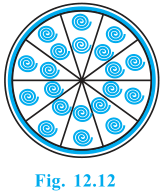Number of diameters = 5
Length of diameter = 35 mm
(i) Total length of silver wire required = Circumference of the circle +  Length of 5 diameter
= 2π r + (5×35) mm = (2 × 22/7 × 35/2) + 175 mm
= 110 + 175 mm = 185 mm

(ii) Number of sectors = 10
Area of each sector = Total area/Number of sectors
Total Area = π r2 = 22/7 × (35/2)2 = 1925/2 mm2
∴ Area of each sector = (1925/2) × 1/10 = 385/4 mm2

10. An umbrella has 8 ribs which are equally spaced (see Fig. 12.13). Assuming umbrella to be a flat circle of radius 45 cm, find the area between the two consecutive ribs of the umbrella.

Number of ribs in umbrella = 8
Radius of umbrella while flat = 45 cm
Area between the two consecutive ribs of the umbrella =
Total area/Number of ribs
Total Area = π r2 = 22/7 × (45)2 = 6364.29 cm2
∴ Area between the two consecutive ribs = 6364.29/8 cm2
= 795.5 cm2

11. A car has two wipers which do not overlap. Each wiper has a blade of length 25 cm sweeping through an angle of 115°. Find the total area cleaned at each sweep of the blades.
Answer : Angle of the sector of circle made by wiper = 115°
Radius of wiper = 25 cm
Area of the sector made by wiper = (115°/360°) × π r2 cm2
= 23/72 × 22/7 × 252 =  23/72 × 22/7 × 625 cm2
= 158125/252 cm2
Total area cleaned at each sweep of the blades = 2 ×158125/252 cm2 =  158125/126 = 1254.96 cm2

12. To warn ships for underwater rocks, a lighthouse spreads a red coloured light over a sector of angle 80° to a distance of 16.5 km. Find the area of the sea over which the ships are warned.

(Use π = 3.14)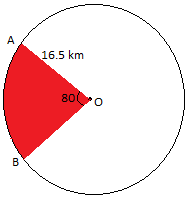Answer : Let O bet the position of Lighthouse.
Angle made by the sector = 80°
Area of the sea over which the ships are warned = Area made by the sector.
Area of sector = (80°/360°) × π r2 km2
= 2/9 × 3.14 × (16.5)2 km2
=  189.97 km2

13. A round table cover has six equal designs as shown in Fig. 12.14. If the radius of the cover is 28 cm, find the cost of making the designs at the rate of ₹ 0.35 per cm2 . (Use √3 = 1.7)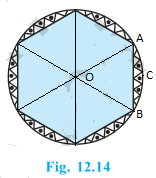Number of equal designs = 6
Radius of round table cover = 28 cm
Cost of making design = ₹ 0.35 per cm2
∠O = 360°/6 = 60°

ΔAOB is isosceles as two sides are equal. (Radius of the circle)
∴ ∠A = ∠B
Sum of all angles of triangle = 180°
∠A + ∠B + ∠O = 180°
⇒ 2 ∠A = 180° – 60°
⇒ ∠A = 120°/2
⇒ ∠A = 60°
Triangle is equilateral as ∠A = ∠B = ∠C = 60°
Area of equilateral ΔAOB = √3/4 × (OA)2 = √3/4 × 282 = 333.2 cm2

Area of sector ACB = (60°/360°) × π r2 cm2
= 1/6 × 22/7 × 28 × 28 = 410.66 cm2
Area of design = Area of sector ACB – Area of equilateral ΔAOB
= 410.66 cm2 – 333.2 cm2 = 77.46 cm2

Area of 6 design = 6 × 77.46 cm2 = 464.76 cm2

Total cost of making design = 464.76 cm2 × ₹ 0.35 per cm2 = ₹ 162.66
14. Tick the correct answer in the following :
Area of a sector of angle p (in degrees) of a circle with radius R is
(A) p/180 × 2πR             (B) p/180 × π R2                   (C) p/360 × 2πR           (D) p/720 × 2πR2
Answer : Area of a sector of angle p = p/360 × π R2
= p/360 × 2/2 × π R2
=  2p/720 × 2πR2
Hence, Option (D) is correct.

Page No: 234
Exercise: 12.3

Unless stated otherwise, use π =22/7
1. Find the area of the shaded region in Fig. 12.19, if PQ = 24 cm, PR = 7 cm and O is the centre of the circle.

PQ = 24 cm and PR = 7 cm
∠P = 90° (Angle in the semi-circle)
∴ QR is hypotenuse of the circle = Diameter of the circle.
By Pythagoras theorem,
QR2 = PR2  + PQ2
⇒ QR2 = 72  + 242
⇒ QR2 = 49  + 576
⇒ QR2 = 625
⇒ QR = 25 cm
∴ Radius of the circle = 25/2 cm
Area of the semicircle = (π R2)/2
= (22/7 × 25/2 × 25/2)/2 cm2
= 13750/56 cm2 = 245.54 cm2
Area of the ΔPQR = 1/2 × PR × PQ
= 1/2 × 7 × 24 cm2
= 84 cm2
Area of the shaded region = 245.54 cm2 – 84 cm2 = 161.54 cm2

2. Find the area of the shaded region in Fig. 12.20, if radii of the two concentric circles with centre O are 7 cm and 14 cm respectively and ∠AOC = 40°.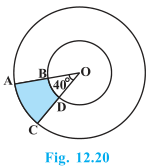Radius of outer circle = 14 cm
Angle made by sector = 40°
Area of the sector OAC = (40°/360°) × π r2 cm2
= 1/9 × 22/7 × 142 = 68.44 cm2
Area of the sector OBD = (40°/360°) × π r2 cm2
= 1/9 × 22/7 × 72  = 17.11 cm2

Area of the shaded region ABDC = Area of the sector OAC – Area of the sector circle OBD

= 68.44 cm2 – 17.11 cm2 = 51.33 cm2

3. Find the area of the shaded region in Fig. 12.21, if ABCD is a square of side 14 cm and APD and BPC are semicircles.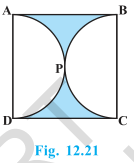Answer : There are two semicircles in the figure.
Side of the square = 14 cm
Diameter of the semicircle = 14 cm
∴ Radius of the semicircle = 7 cm
Area of the square = 14 × 14 = 196 cm2
Area of the semicircle = (π R2)/2

= (22/7 × 7 × 7)/2 cm2 = 77 cm2
Area of two semicircles = 2 × 77 cm2  = 154 cm2
Area of the shaded region = 196 cm2 – 154 cm2 = 42 cm2

4. Find the area of the shaded region in Fig. 12.22, where a circular arc of radius 6 cm has been drawn with vertex O of an equilateral triangle OAB of side 12 cm as centre.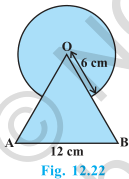Answer : OAB is an equilateral triangle with each angle equal to 60°.
Area of the sector is common in both.
Radius of the circle = 6 cm.
Side of the triangle = 12 cm.
Area of the equilateral triangle = √3/4 × (OA)2 = √3/4 × 122 = 36√3 cm2
Area of the circle = π R2 = 22/7 × 62 = 792/7 cm2

Area of the sector making angle 60° = (60°/360°) × π r2 cm2
= 1/6 × 22/7 × 62 cm2  = 132/7 cm2
Area of the shaded region = Area of the equilateral triangle + Area of the circle – Area of the sector

= 36√3 cm2 + 792/7 cm2 – 132/7 cm2
= (36√3 + 660/7) cm2
5. From each corner of a square of side 4 cm a quadrant of a circle of radius 1 cm is cut and also a circle of diameter 2 cm is cut as shown in Fig. 12.23. Find the area of the remaining portion of the square.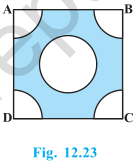Side of the square = 4 cm
Radius of the circle = 1 cm
Four quadrant of a circle are cut from corner and one circle of radius are cut from middle.
Area of square = (side)2 = 42 = 16 cm2
Area of the quadrant = (π R2)/4 cm2 = (22/7 × 12)/4 = 11/14 cm2
∴ Total area of the 4 quadrants = 4 × (11/14) cm2 = 22/7 cm2

Area of the circle = π R2 cm2 = (22/7 × 12) = 22/7 cm2
Area of the shaded region = Area of square – (Area of the 4 quadrants + Area of the circle)

= 16 cm2 – (22/7 + 22/7) cm2
= 68/7 cm2
6. In a circular table cover of radius 32 cm, a design is formed leaving an equilateral triangle ABC in the middle as shown in Fig. 12.24. Find the area of the design.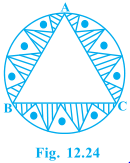Draw a median AD of the triangle passing through the centre of the circle.
⇒ BD = AB/2
Since, AD is the median of the triangle
⇒ 2/3 AD = 32 cm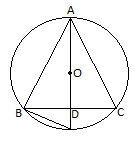By Pythagoras theorem,
⇒ AB2 = 482  + (AB/2)2
⇒ AB2 = 2304  + AB2/4
⇒ 3/4 (AB2) = 2304
⇒ AB2 = 3072
⇒ AB = 32√3 cm
Area of ΔADB = √3/4 × (32√3)2 cm2 = 768√3 cm2
Area of circle = π R2 = 22/7 × 32 × 32 = 22528/7 cm2

Area of the design = Area of circle – Area of ΔADB
= (22528/7 – 768√3) cm2

Page No: 236

7. In Fig. 12.25, ABCD is a square of side 14 cm. With centres A, B, C and D, four circles are drawn such that each circle touch externally two of the remaining three circles. Find the area of the shaded region.

Side of square = 14 cm
Four quadrants are included in the four sides of the square.
∴ Radius of the circles = 14/2 cm = 7 cm
Area of the square ABCD = 142 = 196 cm2
Area of the quadrant = (π R2)/4 cm2 = (22/7 × 72)/4 cm2

= 77/2 cm2
Total area of the quadrant = 4 × 77/2 cm2 = 154 cm2
Area of the shaded region = Area of the square ABCD – Area of the quadrant
= 196 cm2 – 154 cm2
= 42 cm2
8. Fig. 12.26 depicts a racing track whose left and right ends are semicircular.

The distance between the two inner parallel line segments is 60 m and they are each 106 m long. If
the track is 10 m wide, find :
(i) the distance around the track along its inner edge
(ii) the area of the track.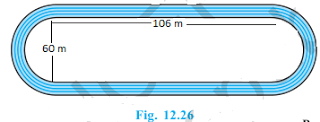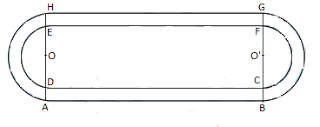Answer : Width of track = 10 m
Distance between two parallel lines = 60 m
Length of parallel tracks = 106 m
DE = CF = 60 m
Radius of inner semicircle, r = OD = O’C
= 60/2 m = 30 m
Radius of outer semicircle, R = OA = O’B
= 30 + 10 m = 40 m
Also, AB = CD = EF = GH = 106 m
Distance around the track along its inner edge = CD + EF + 2 × (Circumference of inner semicircle)
= 106 + 106 + (2 × πr) m = 212 + (2 × 22/7 × 30) m
= 212 + 1320/7 m = 2804/7 m
Area of the track = Area of ABCD + Area EFGH + 2 × (area of  outer semicircle) – 2 × (area of  inner                                                                                                                                               semicircle)
= (AB × CD) + (EF × GH) + 2 × (πr2/2) – 2 × (πR2/2) m2
= (106 × 10) + (106 × 10) + 2 × π/2 (r2 -R2) m2
= 2120 + 22/7 × 70 × 10 m2
= 4320 m2
9. In Fig. 12.27, AB and CD are two diameters of a circle (with centre O) perpendicular to each other
and OD is the diameter of the smaller circle. If OA = 7 cm, find the area of the shaded region.

Radius of larger circle, R = 7 cm
Radius of smaller circle, r = 7/2 cm
Height of ΔBCA = OC = 7 cm
Base of ΔBCA = AB = 14 cm
Area of ΔBCA = 1/2 × AB × OC = 1/2 × 7 × 14 = 49 cm2
Area of larger circle = πR2 = 22/7 × 72 = 154 cm2

Area of larger semicircle = 154/2 cm2 = 77 cm2

Area of smaller circle = πr2 = 22/7 × 7/2 × 7/2 = 77/2 cm2

Area of the shaded region = Area of larger circle – Area of triangle – Area of larger semicircle + Area                                               of smaller circle

Area of the shaded region = (154 – 49 – 77 + 77/2) cm2
= 133/2 cm2 = 66.5 cm2
10. The area of an equilateral triangle ABC is 17320.5 cm2. With each vertex of the triangle as centre, a circle is drawn with radius equal to half the length of the side of the triangle (see Fig. 12.28). Find the area of the shaded region. (Use π = 3.14 and √3 = 1.73205)
ABC is an equilateral triangle.
∴ ∠A = ∠B = ∠C = 60°
There are three sectors each making 60°.
Area of ΔABC = 17320.5 cm2
⇒ √3/4 × (side)2  = 17320.5
⇒ (side)2  = 17320.5 × 4/1.73205
⇒ (side)2  = 4 × 104
⇒ side  = 200 cm
Radius of the circles = 200/2 cm = 100 cm
Area of the sector = (60°/360°) × π r2 cm2
= 1/6 × 3.14 × (100)2 cm2
= 15700/3 cm2
Area of 3 sectors = 3 × 15700/3 = 15700 cm2 =
Area of the shaded region = Area of equilateral triangle ABC – Area of 3 sectors
= 17320.5 – 15700 cm2 = 1620.5 cm2

11. On a square handkerchief, nine circular designs each of radius 7 cm are made (see Fig. 12.29). Find the area of the remaining portion of the handkerchief.

Number of circular design = 9
Radius of the circular design = 7 cm
There are three circles in one side of square handkerchief.
∴ Side of the square = 3 × diameter of circle = 3 × 14 = 42 cm
Area of the square = 42 × 42 cm2 = 1764 cm2
Area of the circle = π r2 = 22/7 × 7 × 7 = 154 cm2

Total area of the design = 9 × 154 = 1386 cm2
Area of the remaining portion of the handkerchief = Area of the square – Total area of the design
= 1764 – 1386 = 378 cm2
12. In Fig. 12.30, OACB is a quadrant of a circle with centre O and radius 3.5 cm. If OD = 2 cm, find the area of the

(i) Area of quadrant OACB = (πR2)/4 cm2
= (22/7 × 7/2 × 7/2)/4 cm2
= 77/8 cm2
(ii) Area of triangle BOD = 1/2 × 7/2 × 2 cm2

= 7/2 cm2
Area of shaded region = Area of quadrant – Area of triangle BOD

= (77/8 – 7/2) cm2 = 49/8 cm2
= 6.125 cm2

13. In Fig. 12.31, a square OABC is inscribed in a quadrant OPBQ. If OA = 20 cm, find the area of the shaded region. (Use π = 3.14)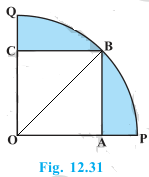Answer : Side of square = OA = AB = 20 cm
OAB is right angled triangle
By Pythagoras theorem in ΔOAB ,
OB2 = AB2  + OA2
⇒ OB2 = 202  + 202
⇒ OB2 = 400  + 400
⇒ OB2 = 800
⇒ OB = 20√2 cm
Area of the quadrant = (πR2)/4 cm2 = 3.14/4 × (20√2)2 cm2 = 628 cm2
Area of the square = 20 × 20 = 400  cm2

Area of the shaded region = Area of the quadrant – Area of the square

= 628 – 400 cm2 = 228 cm2

14. AB and CD are respectively arcs of two concentric circles of radii 21 cm and 7 cm and centre O (see Fig. 12.32). If ∠AOB = 30°, find the area of the shaded region.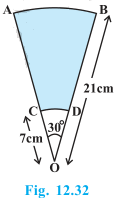Radius of the smaller circle, r = 7 cm
Angle made by sectors of both concentric circles =  30°
Area of the larger sector = (30°/360°) × π R2 cm2
= 1/12 × 22/7 × 212 cm2
= 231/2 cm2
Area of the smaller circle = (30°/360°) × π r2 cm2

= 1/12 × 22/7 × 72 cm2
= 77/6 cm2
Area of the shaded region = 231/2 – 77/6 cm2

= 616/6 cm2 = 308/3 cm2

15. In Fig. 12.33, ABC is a quadrant of a circle of radius 14 cm and a semicircle is drawn with BC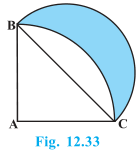as diameter. Find the area of the shaded region.
AB = AC = 14 cm
BC is diameter of semicircle.
ABC is right angled triangle.
By Pythagoras theorem in ΔABC,
BC2 = AB2  + AC2
⇒ BC2 = 142  + 142
⇒ BC = 14√2 cm
Radius of semicircle = 14√2/2 cm = 7√2 cm
Area of ΔABC = 1/2 × 14 × 14 = 98 cm2
Area of quadrant = 1/4 × 22/7 × 14 × 14 = 154 cm2

Area of the semicircle = 1/2 × 22/7 × 7√2 × 7√2 = 154 cm2

Area of the shaded region = Area of the semicircle + Area of ΔABC – Area of quadrant
= 154 + 98 – 154 cm2 = 98 cm2
Page No: 238

16. Calculate the area of the designed region in Fig. 12.34 common between the two quadrants of circles of radius 8 cm each.